## Introduction to the Economic Value Added (EVA)

The EVA (Economic Value Added) is an indicator of profitability and a measure of financial performance, based on residual wealth. It is the excess profit above the cost of capital, generated by the business, adjusted for taxes, and presented on a cash basis. The consulting firm Stern Value Management developed the method. It represents the difference between the Rate of Return and Cost of Capital and measures the value generated by invested capital.

The Economic Value Added (EVA) attempts to capture the truest economic profitability of the company. Therefore, we also refer to it as Economic Profit.

A negative EVA means that the business is generating no value. Whereas, a positive EVA implies the company is creating value for the shareholders.

Financial analysts rely on various methods to measure the value a business or project generates. Return on Invested Capital (ROIC) is a standard method that also uses a residual income approach. However, most financial analysts consider the best measure to be the value of generated cash flows, which can be measured by the Internal Rate of Return (IRR). The IRR is used extensively in financial modeling to capture all aspects of the performance of the business or project.

## How to calculate the Economic Value Added (EVA)

We calculate the EVA via the following formula:Where:

When we can’t find the Invested capital, we can modify the formula as follows: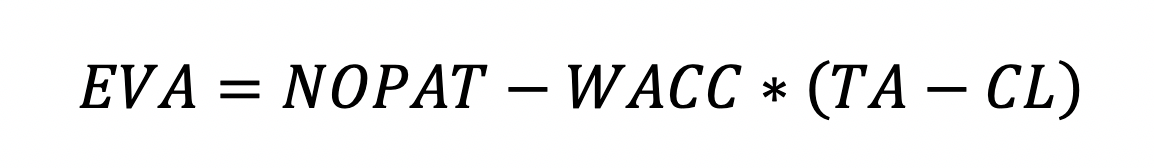Where:

• TA represnets the Total Assets from the balance sheet;
• CL represents the Current Liabilities from the balance sheet.

EVA aims to quantify the cost of investing capital into a business or project. It also aims to assess whether the business or project generates enough cash returns to be considered a good investment. A positive EVA means that the project is making profits above the required minimum return. The EVA looks at businesses from the perspective that a company or project is only profitable when it creates wealth and returns for the shareholders.

Looking at EVA from the perspective of Return on Invested Capital (ROIC), we can approach the calculation with the following formula: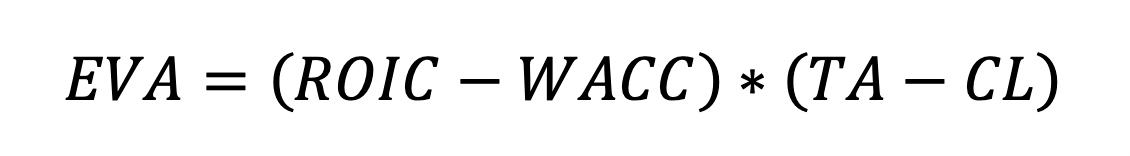We can calculate ROIC via the equation: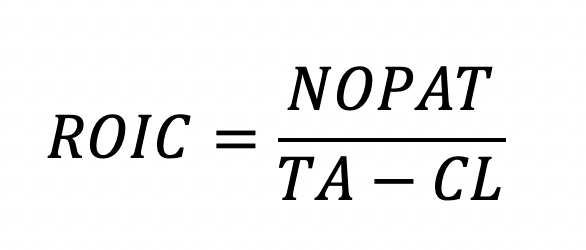Replacing ROIC in the above formula and extending the brackets, we arrive at the same representation for EVA: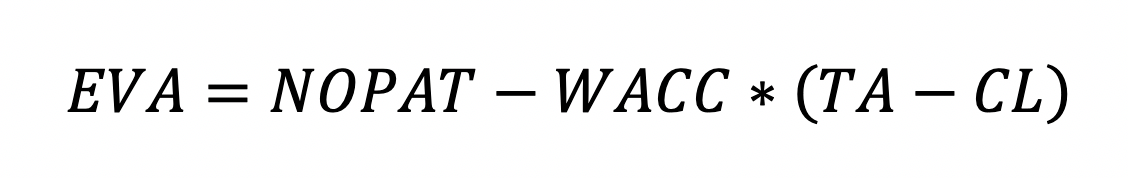As (Total Assets – Current Liabilities) is the invested capital, we can further present the formula as:where c is the cost of capital.

The Net Operating Profit After Tax can be presented as function of invested capital as well, thus giving us the formula: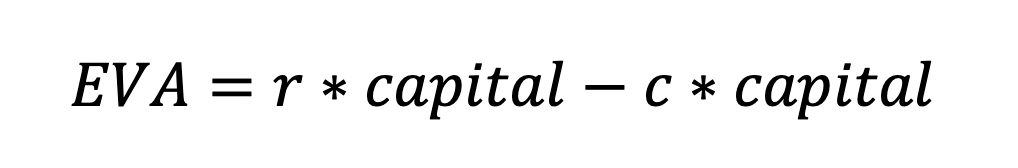where r is the rate of return, and ultimately leading us to: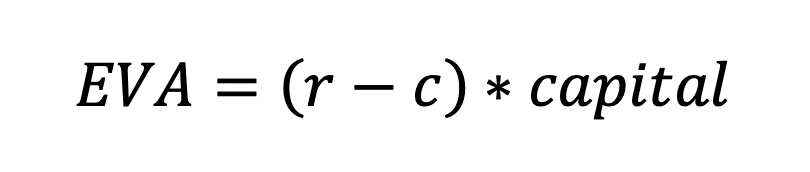## Net Operating Profit After Tax (NOPAT)

A key consideration when calculating the Net Operating Profit After Tax (NOPAT) is the adjustment of the interest cost. The reason is that the finance charge includes the interest charge, which we deduct from NOPAT. There are two approaches to calculating NOPAT:

1. Operating profit includes the tax benefit from the interest charge. Therefore, when approaching NOPAT from the Operating profit, we deduct the adjusted tax charge. To achieve this, we multiply the interest by the tax rate and add it back to the tax charge.
2. The more straightforward approach is to start with profit after tax and add back the net cost of interest. To achieve this, we multiply the interest charge by the tax shield (1 – tax rate).

## Finance Charge in the EVA calculation

We also refer to the multiple of the Weighted Average Cost of Capital (WACC) and the Invested Capital from the EVA formula as the Finance Charge.

Usually, we calculate the Invested Capital by adding together the value of total Equity and the amount of any long-term debt balances at the beginning of the period under observation.

When preparing our inputs to the Economic Value Added calculation, we need to add back all provisions, doubtful debt allowances (DDA), inventory allowances, and deferred tax provisions to the invested capital.

We also add back non-cash expenses to profit and invested capital.

If the company has operating leases, we present those as financial leases, meaning we capitalize them and add them to capital employed.

In contradiction with the accrual basis, on which we base financial reporting, we calculate the tax charge on the cash basis for the EVA calculation: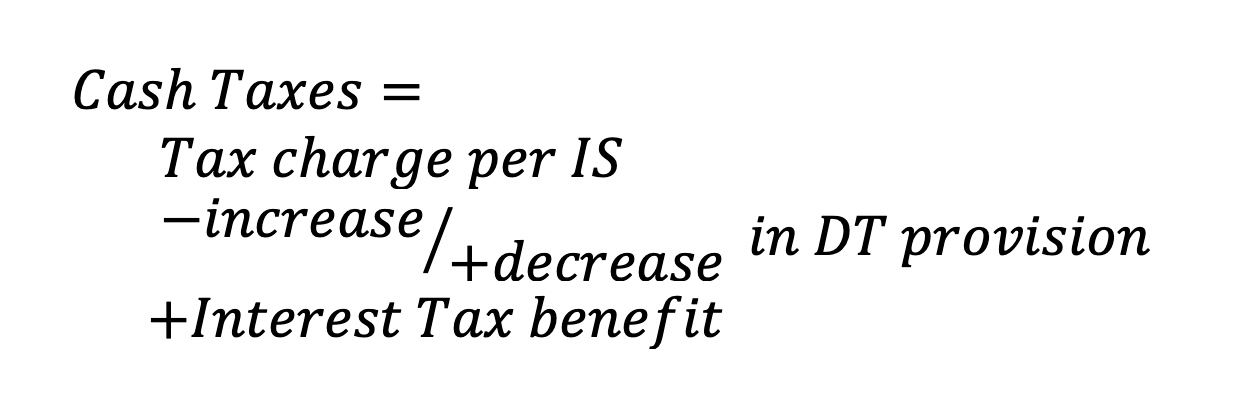• First, we subtract the increase or add the decrease in the Deferred Tax provisions;
• Then, we add the tax benefit on the interest costs.

The Market Value Added (MVA) is an associated metric to the EVA calculation. It shows us the total value an investment creates for its shareholders from the total invested capital over the entire investment period. We calculate the MVA by summing the discounted present values of all expected future EVA’s.

We can present the calculation with the following formula:The Economic Value Added (EVA) is a handy performance indicator as the calculation shows where the business created wealth via balance sheet items. That way, the EVA approach forces management to be more responsible for assets and expenses while making decisions.

The calculation relies heavily on the amount of invested capital and is therefore suitable for asset-rich companies that have matured and have stable performance. Companies with substantial intangible assets, like IT businesses, are usually not good candidates for EVA.

In more complex business scenarios cash adjustments can pile-up seemingly to infinity. As a result, the EVA calculation may become too complicated and time-consuming.

Accrual distortions can also affect the Economic Value Added, primarily depreciation and amortization differences.

One of the most significant minuses for the EVA calculation is that it looks only at the measured period and is not predictive of future performance.

## Compare the Economic Value Added (EVA) to other approaches

To get a better understanding of where EVA fits in financial analysis, let us compare it to two different methods:

• Discounted Economic Profit
• Measure used: Economic Value Added
• Discount rate: Weighted Average Cost of Capital
• Used for: highlights when a company is generating a rate of return above its cost of capital
• Enterprise Discounted Cash Flows
• Measure used: Free Cash Flow
• Discount rate: Unlevered Cost of Capital
• Usage: highlights capital structure changes more quickly than WACC-based models.

## Example EVA calculation

To illustrate the concept behind the Economic Value Added (EVA), let us look at how the calculation might be performed in a simple scenario. To calculate it we need the effective tax rate and the operating profit, which we can get from the Income Statement of the company.

Assuming that there are no specific adjustments to be taken, we can calculate NOPAT like that:Next value we need is the Invested Capital. We calculate it by adding the Equity of the Company and it’s Long-term Debt. These we can get from the public Balance Sheet or Statement of Financial Position.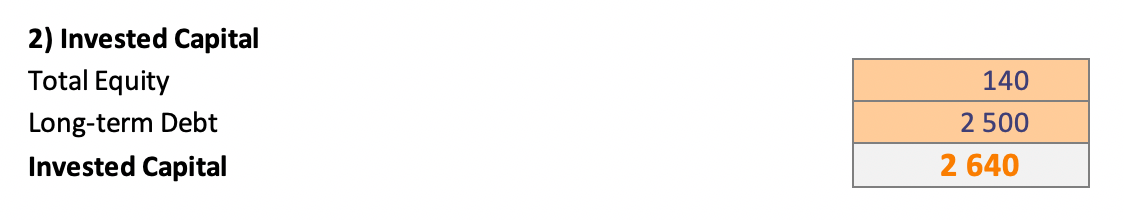Having the NOPAT and the Invested Capital, we only need to calculate the Weighted Average Cost of Capital (WACC). We will not go into too much detail here, as we have already covered WACC in a separate article, that you can read here.Now that we have all required elements to calculate the EVA, we can do so, by following the above discussed formula:The positive value implies that this business is one that generates wealth for the shareholders and is a good investment.

## Conclusion

The Economic Value Added is an important metric in financial modeling and analysis. We use it as an indicator of how profitable projects are and it serves as a reflector of management performance. The idea behind the EVA is that businesses are only profitable when they create wealth for their shareholders, and the measure for this goes beyond net income. The EVA calculation aims to make sure the business generates returns above its cost of capital.## FCCA, FMVA

Hi! I am a finance professional with 10+ years of experience in audit, controlling, reporting, financial analysis and modeling. I am excited to delve deep into specifics of various industries, where I can identify the best solutions for clients I work with.

In my spare time, I am into skiing, hiking and running. I am also active on Instagram and YouTube, where I try different ways to express my creative side.

The information and views set out in this publication are those of the author(s) and do not necessarily reflect the official opinion of Magnimetrics. Neither Magnimetrics nor any person acting on their behalf may be held responsible for the use which may be made of the information contained herein. The information in this article is for educational purposes only and should not be treated as professional advice. Magnimetrics and the author of this publication accept no responsibility for any damages or losses sustained in the result of using the information presented in the publication.